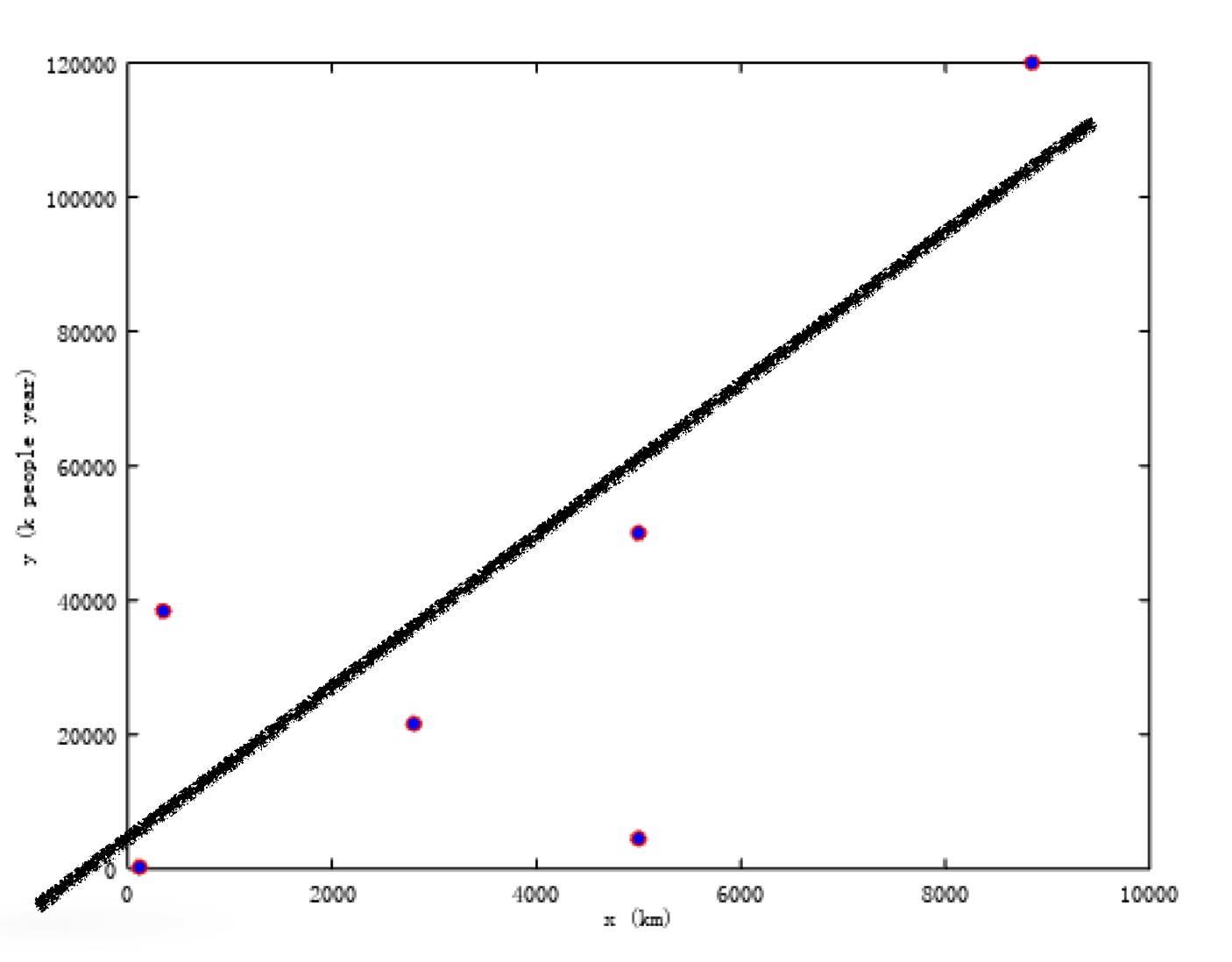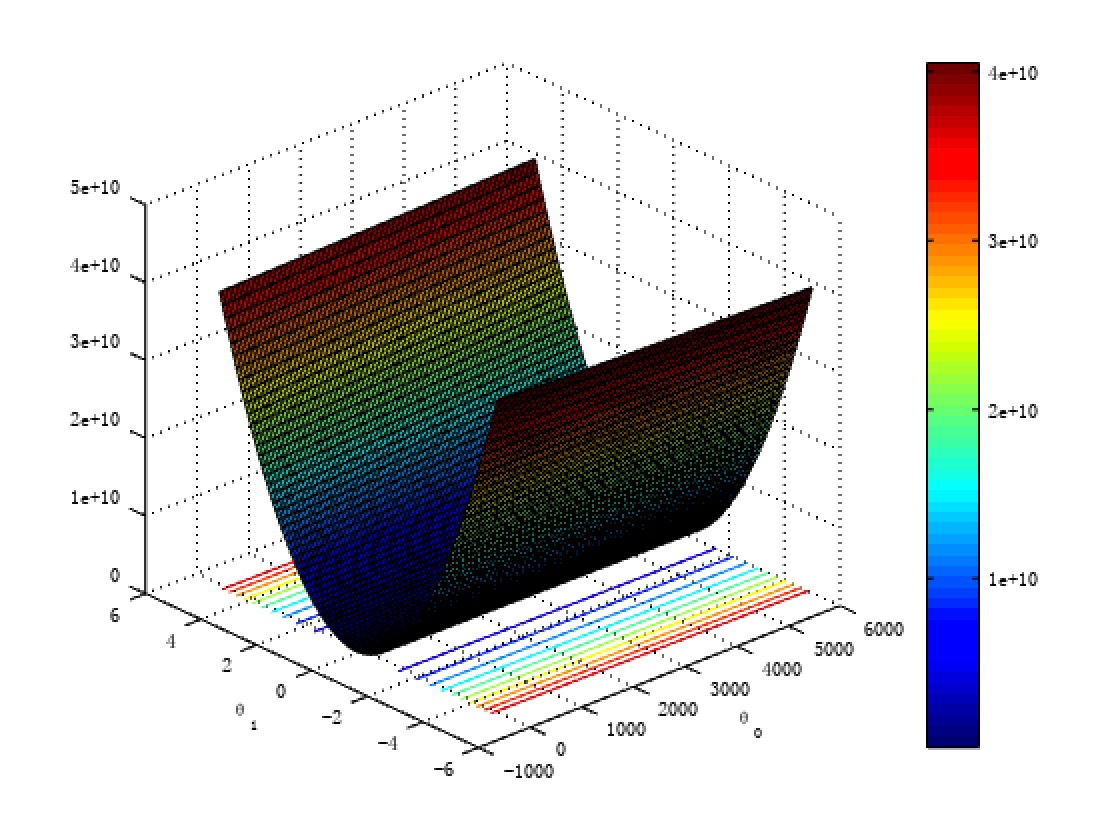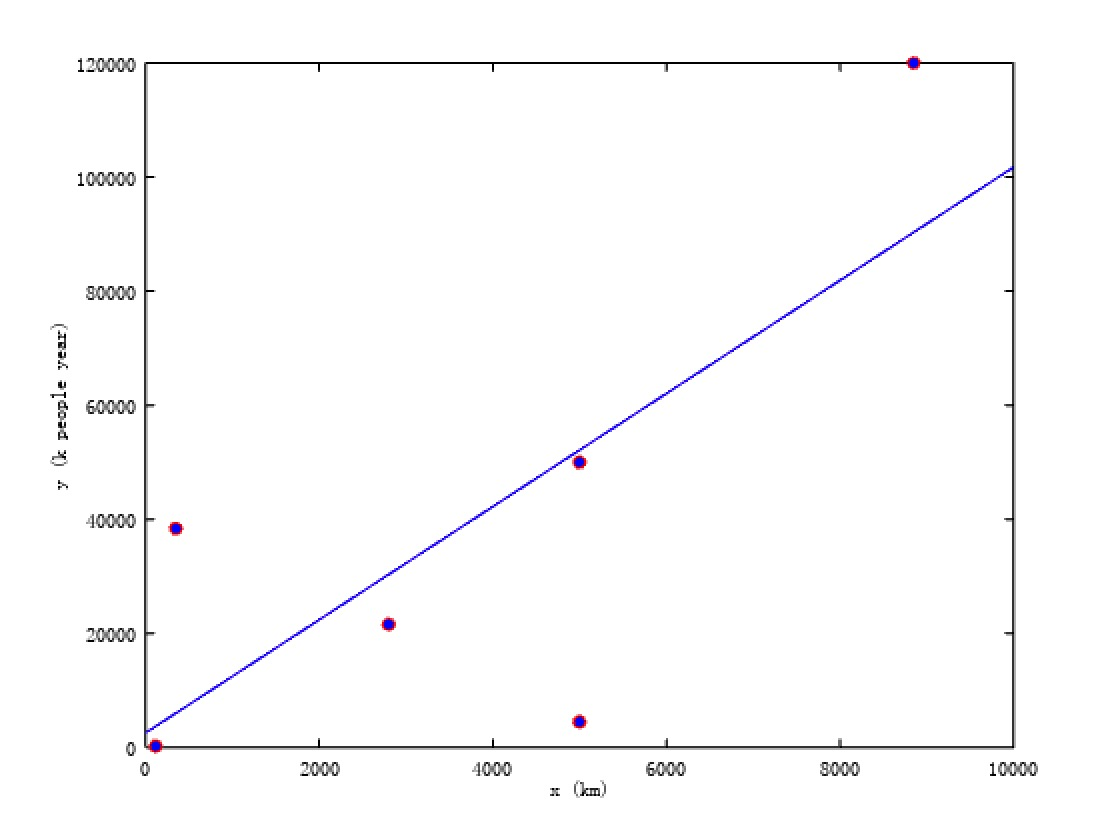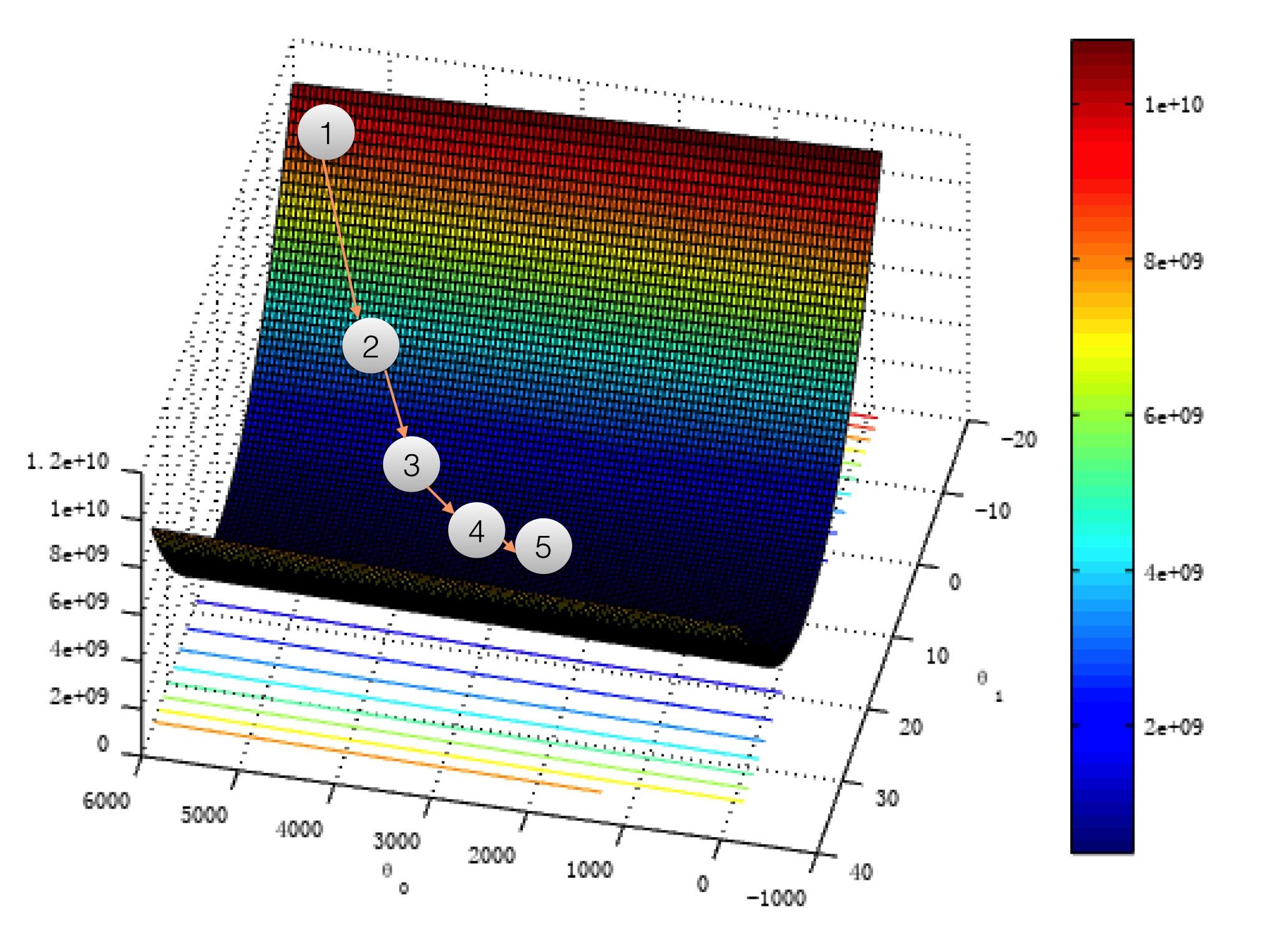### Introduction

In the end of 2016, Trump occupied the presidency. We are always thinking about how he will build the wall between US and Mexico, Today, I’d like to compute how many resources he would cost if he truly began to build the wall using linear regression.

### Data

The table below is some basic information about Chinese GreatWall as well as one item about Hadrian’s Wall：

Age people(1000) Years Length(KM)
Qin Dynasty 300 15 5000
Han Dynasty 500 100 5000
North Northern Dynasties 1800 12 2800
Sui Dynasty 1280 30 350
Ming Dynasty 3000 40 885

We use matrices represent the resources and the length of these walls. Each row of $x$ represents the quantity of (1000 people and year) men and years, and each row of $y$ denotes the length of walls(KiloMeter)

\begin{align}
X=\begin{bmatrix}
5000\
5000\
2800\
350\
8851\
117
\end{bmatrix}
y=
\begin{bmatrix}
300*15\
500*100\
1800*12\
1280*30\
3000*40\
18*14
\end{bmatrix}
\end{align}

Let’s draw the picture of these data:We hope find a mapping $x\rightarrow f(x)$ ($x$ denotes the length of walls, $y$ denotes the cost of resources)drawing a line as the figure above which meets all data best. When we encounter new data(e.g. new length of wall), we hope $f(x)$ will help us find how many resources will we cost. This is the goal of linear regression.

### Definition

First of all, we assume that the line have the form as followed:
$$h_{\theta}(x)=\theta_0+\theta_1 x_1+\theta_2 x_2 + \cdots + \theta_nx_n$$
Specifically, under our circumstance, there is only one $x$(the length of walls), then we will have the form as below:
$$h_{\theta}(x)=\theta_0+\theta_1x$$
In fact, there are many factor infulence the outcome of the cost of wall besides people and time. Tools we use and the economy as well as terrain where building the wall. if we add these factors, maybe the assumption looks like:
$$h_{\theta}(x)=\theta_0+\theta_1x_1+\theta_2x_2+\theta_3x_2$$
$x_1$denotes the length of wall, while $x_2$ and $x_3$ denote economy and terrian condition respectively. For simplicity, we consider only the length of wall.

In general, we add a $x_0=1$ to the equation, the we have:
$$h_{\theta}(x)=\theta_0x_0+\theta_1 x_1 + \cdots + \theta_nx_n =\sum_{i=1}^{n}\theta_ix_i$$
If we use matrices represent the equation, then
\begin{align}
\Theta=\begin{bmatrix}
\theta_0\
\theta_1\
\cdots\
\theta_n
\end{bmatrix}
\end{align}
thus,
\begin{align}
h_{\theta}(x)=\sum_{i=1}^{n}\theta_ix_i=
\begin{bmatrix}
\theta_0\theta_1\cdots\theta_n
\end{bmatrix}
\begin{bmatrix}
x_0\
x_1\
\cdots\
x_n
\end{bmatrix}
=\Theta^T\overrightarrow{X}
\end{align}
Notice that $\Theta^T$ is a $1\times n$ matrices while $\overrightarrow{X}$ is a $n\times1$ matrices. We get a real number after multiply two matrices.

### Cost Function

We hope find a way to find the best function $h_\theta(x)$ fit these data. Notice that if $|(h_\theta(x_i)-y_i)|=0$ for each $x$, then the function fit data absolutely. In practice, if we minimize $|(h_\theta(x_i)-y_i)|$, we can find the best fit to original data. An optional cost function is square cost function:
$$J(\theta)=\frac{1}{2m}\sum_{i=1}^{m}(h_\theta(x_i)-y_i)^2$$
As long as we can minimize $J(\theta)$ with respect to $\theta$, can we find the best $\theta$ fit original data. The cost function looks like as followed:The figure has a bowl shape, and we can find the minimum of it both using matrices approach and gradient descent.

### Matrics Solution

Remember that the cost function $J(\theta)=\frac{1}{2m}\sum_{i=1}^{m}(h_\theta(x_i)-y_i)^2$, It is more convinient if we apply $J(\theta)$ matrics form, then:
\begin{align}
J(\theta)=\frac{1}{2m}(h_\theta(x)-y)^T\cdot(h_\theta(x)-y)\
=\frac{1}{2m}(X\Theta-y)^T\cdot(X\Theta-y)
\end{align}
We can take the derivative of $J(\theta)$ and let it be $\overrightarrow{0}$. It is a little complex if we take the derivative of the last equation about $J(\theta)$. Let’s do the derivation a easy way, but not a total Matric solution. If we do the derivation on the sum, we can get:
$$\frac{\partial}{\partial\theta_j}J(\theta)=\frac{1}{m}\sum_{i=1}^m(h_\theta(x_i)-y_i)x_j$$
Then,
\begin{align}
\frac{\partial}{\partial\Theta}J(\theta)=
\begin{bmatrix}
\frac{\partial}{\partial\theta_1}J(\theta)\
\frac{\partial}{\partial\theta_2}J(\theta)\
\frac{\partial}{\partial\theta_3}J(\theta)\
\cdots\
\frac{\partial}{\partial\theta_n}J(\theta)
\end{bmatrix}=\overrightarrow{0}
\end{align}
Now, we transfer the sum into matrices form:
$$\frac{\partial}{\partial\Theta}J(\theta)=X^T(X\Theta-y)=X^TX\Theta-X^Ty=\overrightarrow{0}$$
thus,
we can get the solution of $\Theta$:
$$\Theta=(X^TX)^{(-1)}X^Ty$$
Let’s look at the solution of Matrices:And $\theta_0$ and $\theta_1$ is as followed:
\begin{align}
\Theta=\begin{bmatrix}
2573\
9.9\
\end{bmatrix}
\end{align}
That means $h_\theta(x)=2573+9.9x$, Right Now we subsitute 3169(km) for $x$:
$$h_\theta(3169)=2573+9.9*3169=33946(k\ people\ year)$$
In other word, Donald Trump need 3,394,600 people continously build 10 years in order to finish the GreatWall between US and Mexico. Surely he can stimulate 33,946,000 people, it only take him one year to finished the wall.

Let’s look at the cost function one more time. If we first choose a random $\Theta$, say we have $\theta_0\&\theta_1$ located in point 1. Assume you stand at ponit 1, you want to find a way to go down the vally. Surely you can not see the land-form completely. But you can look around at point 1 and find a steepest direction, then you go that way a baby step. Right now, you are at point 2 after the first step. You do the same find a steepest direction and make another baby step. After several steps, you are now standing at point 5. When you are looking around you find that where you are standing is almost flat. Now we can stop the iteration, and we have found a reasonable $\Theta$ which makes the $J(\theta)$ has a minmum value.What I described is a method named Gradient Descent which is very easy way to find minima of $J(\theta)$. The step is as followed:

1. Find a reasonable cost function J(\theta).
2. Take the partial derivative of $J(\theta)$ for each $\theta_j$
3. Choose a moderate $\alpha$ to constrain the step size.
4. Take a baby step, the direction is from the derivative and the size is determined by derivative and parameter $alpha$
5. update the value of $\Theta$, check if we find the minimum. If not, return to the step 4, otherwise, stop the algo

Concretely, the algorithm using octave is listed below:

Under the circumstance above, $\theta_0=0.0048$ and $\theta_1=10.33$. If we substitute the parameters for $h_\theta(x)$, the answer that Trump would take to build the wall is 32745.56$\times$1000 people year.

### Summarize

Today we have talk about how to use linear regression to model a problem of building Great Wall. We have also talk about how to resovle the problem through minimize the cost function both using Matrices solution and Gradient Descent.